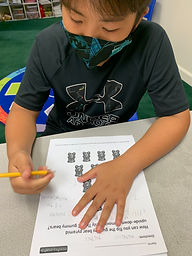Ms. Kayla

Target 1​

Lesson Type:

Review

Critical Thinking

:

Logical Reasoning

Use reasoning to solve logic puzzles.

1:

Use the given information in a problem to identify the correct answer.

2:

Follow given rules to solve problems.

5th

Vocabulary:

Theory, Pattern, Sequence, Rule, Rotate

Activities:

1. Student had to move the objects to rotate the image 180 degress.

2. Student then worked backwards to determine a pattern and worked on additional puzzles to test out the pattern theory.Home Exploration

Guiding Questions:Absent Students:

Target 2

:

1:

Utilize the standard algorithm to divide fractions.

2:

With two fractions, find the reciprocal of the 2nd fraction, replace the ÷ with x, multiply numerators and denominators, simplify.

3:

With a whole number by a fraction, convert the whole number to an improper fraction, find the reciprocal of the 2nd fraction, replace the ÷ with x, multiply numerators and denominators, simplify.

4:

With a fraction by a whole number, convert the whole number to improper fraction, find the reciprocal of the 2nd number, replace the ÷ with x, multiply the numerators and denominators, simplify.

5th

Vocabulary:

Mixed Number, Improper Fraction, Division, Multiply, Convert, Simplest form, Numerator, Denominator, Inverse

Activities:

1. Student practiced dividing and multiplying fractions that involved both mixed and improper fractions.

2. Student was taught to use multiplication (inverse) to solve a problem that required the division of fractions.

3. Student was shown a different method of reducing a fraction to its simplest form by divisibility of either the numerator or denominator of the corresponding fraction.Home Exploration

3 2/3 divided by 7/8

What would be your first step to solve this problem?

What is the inverse of division?

Guiding Questions:Target 3

:

Vocabulary:

Activities:Home Exploration